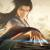一个有趣的算法,实现色块的交换，怎么实现呢

iShown 发布于 2016/02/02 12:41

There're 7 red tiles, 8 blue titles and one white title in a 4 x 4 plane. We could only move the white tile. When moving it, the white tile swaps the position with the adjacent tile. L, R, U, D are corresponding to four directions which the tile could be moved to (Left, Right, Up, Down) For example, starting from configuration (S), by the move sequence RDRDL we reach the configuration (E). Now, starting from configuration (S), find the shortest way to reach configuration (T).

(S)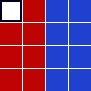(E)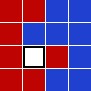(T)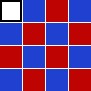What is the move sequence of the path ?

0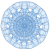```#include <iostream>
#include <cstdlib>
#include <cstring>
#include <vector>
using namespace std;
typedef long state;
//状态压缩，低0~15位：0代表红块或者空格，1代表蓝块
//16~17位：空格x，18~19：空格y

const int dx[]={0,0,1,-1};
const int dy[]={1,-1,0,0};

inline int getX(state s)
{
return (s & ((1<<16) + (1<<17)))>>16;
}

inline int getY(state s)
{
return (s & ((1<<18) + (1<<19)))>>18;
}

int d[1<<20]; //从初始状态到需要多少步
state last[1<<20];//上一步状态是多少

inline bool legal(int x, int y)
{
return x>=0 && x<4 && y>=0 && y<4;
}

inline state xy2bit(int x, int y)
{
return ((x<<2) + y);
}

inline int getBit(state s, state bit)
{
bit = 1<<bit;
return (s & bit) >0 ;
}

state change(state olds, int pdx, int pdy) //给定当前状态和移动的方向，求下一个状态
{
int oldx = getX(olds), oldy = getY(olds);
olds = (olds & 0xffff) + ((oldx + pdx)<<16) + ((oldy+pdy)<<18);
int w = getBit(olds, xy2bit(oldx, oldy)), ww = getBit(olds, xy2bit(oldx+pdx, oldy+pdy));
olds -= (w* (1<<xy2bit(oldx, oldy)) + ww*(1<<xy2bit(oldx+pdx, oldy+pdy)));
olds += (ww* (1<<xy2bit(oldx, oldy)) + w*(1<<xy2bit(oldx+pdx, oldy+pdy)));
return olds;
}

void search(state s)
{
int x=getX(s), y=getY(s);
for (int i=0; i<4; ++i)
if (legal(x+dx[i], y+dy[i]))
{
int news = change(s, dx[i], dy[i]);
if (d[news] == d)
{
last[news] = s;
d[news] = d[s] + 1;
search(news);
}
}
}

vector<pair<int,int> > result;
int main()
{
memset(d, 0x3f, sizeof(d)); // 0x3f3f3f3f: 一个正大数
d[0xcccc] = 0; //0000 1100 1100 1100 1100 初始状态
search(0xcccc);
cout << d[0x5a5a] << endl; // 0000 0101 1010 0101 1010
int s = 0x5a5a;
do
{
result.push_back(make_pair(getX(s), getY(s)));
s = last[s];
} while (d[s] > 0);
result.push_back(make_pair(0, 0));
for (size_t i=0; i<result.size(); ++i)
{
int index = result.size() - 1 -i;
cout << i <<".\t" << result[index].first << " "<< result[index].second << endl;
}

}```

0